Ex 1.3

Chapter 1 Class 11 Sets (Term 1)
Serial order wise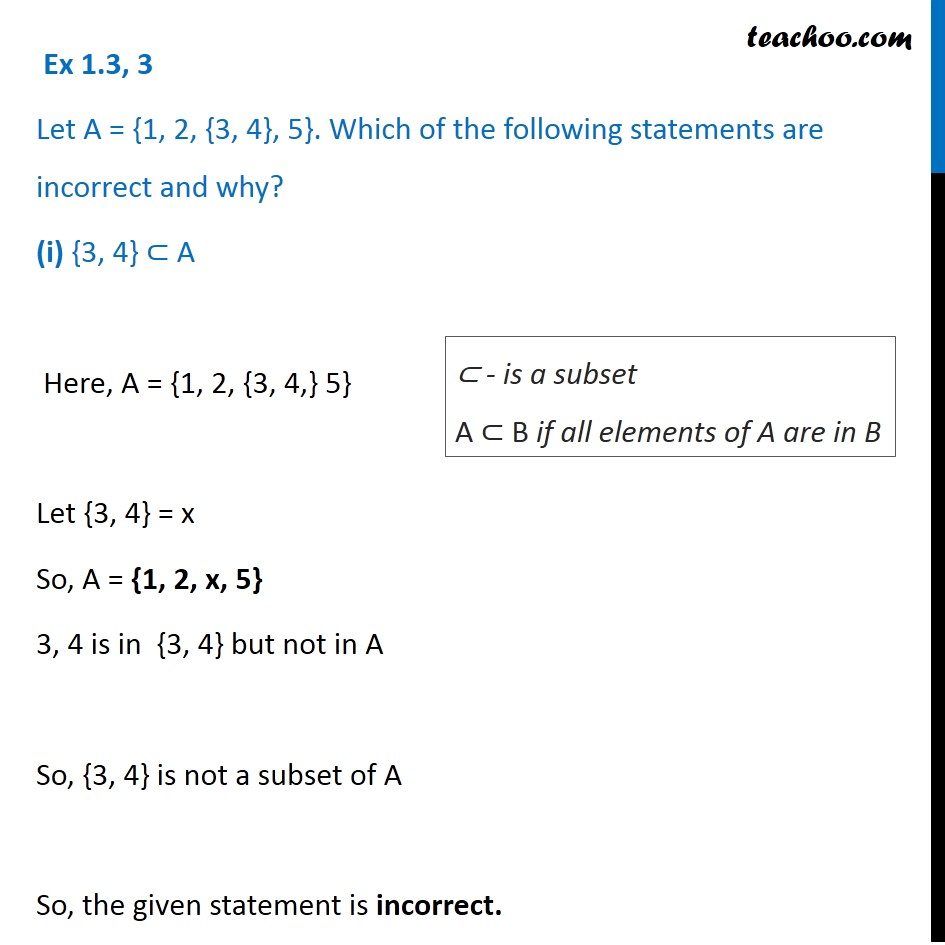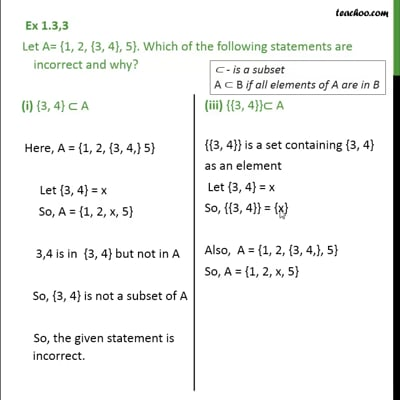This video is only available for Teachoo black users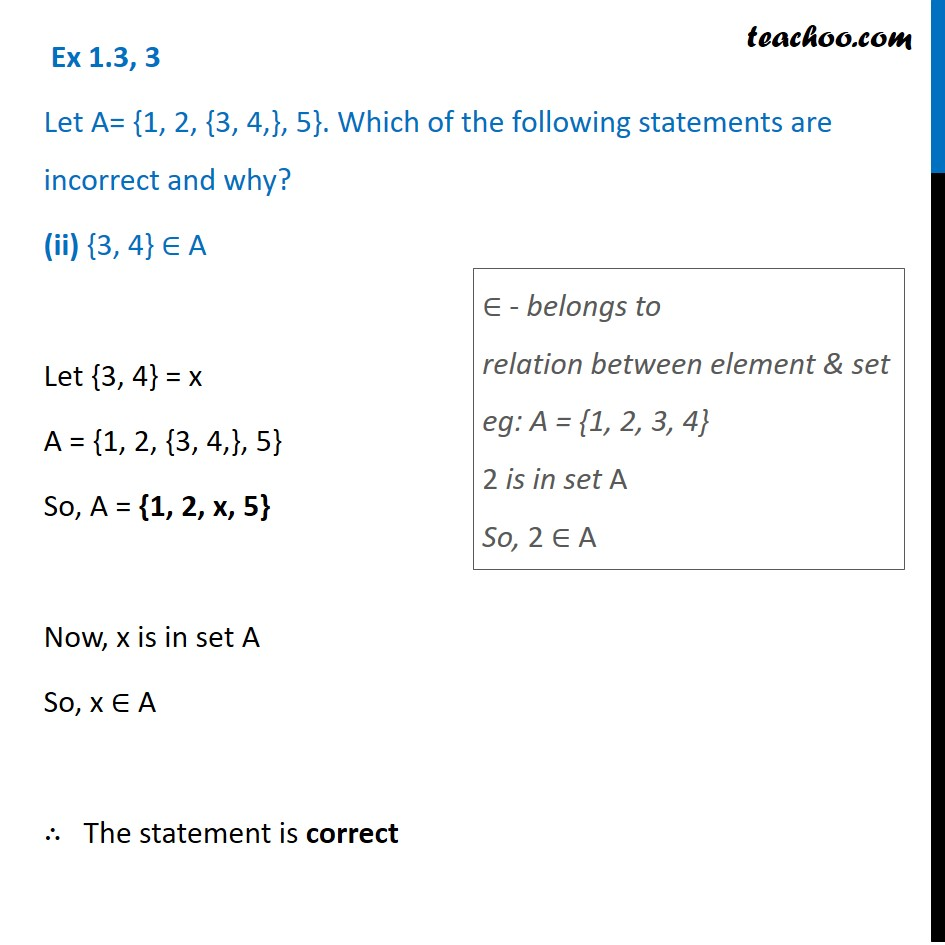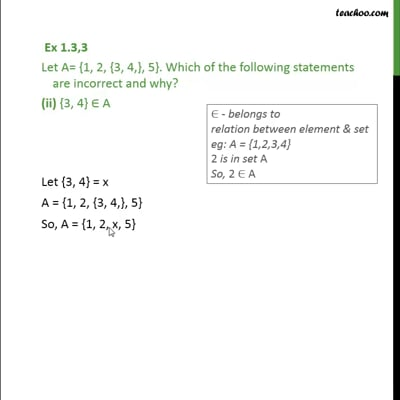This video is only available for Teachoo black users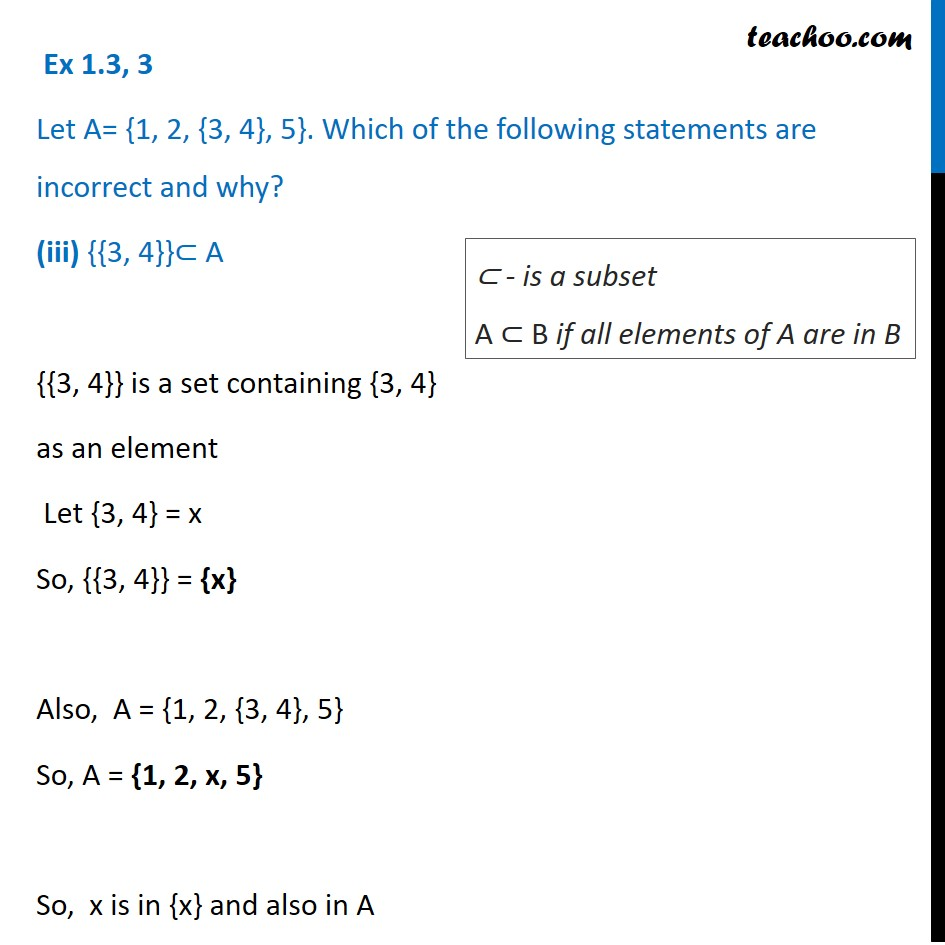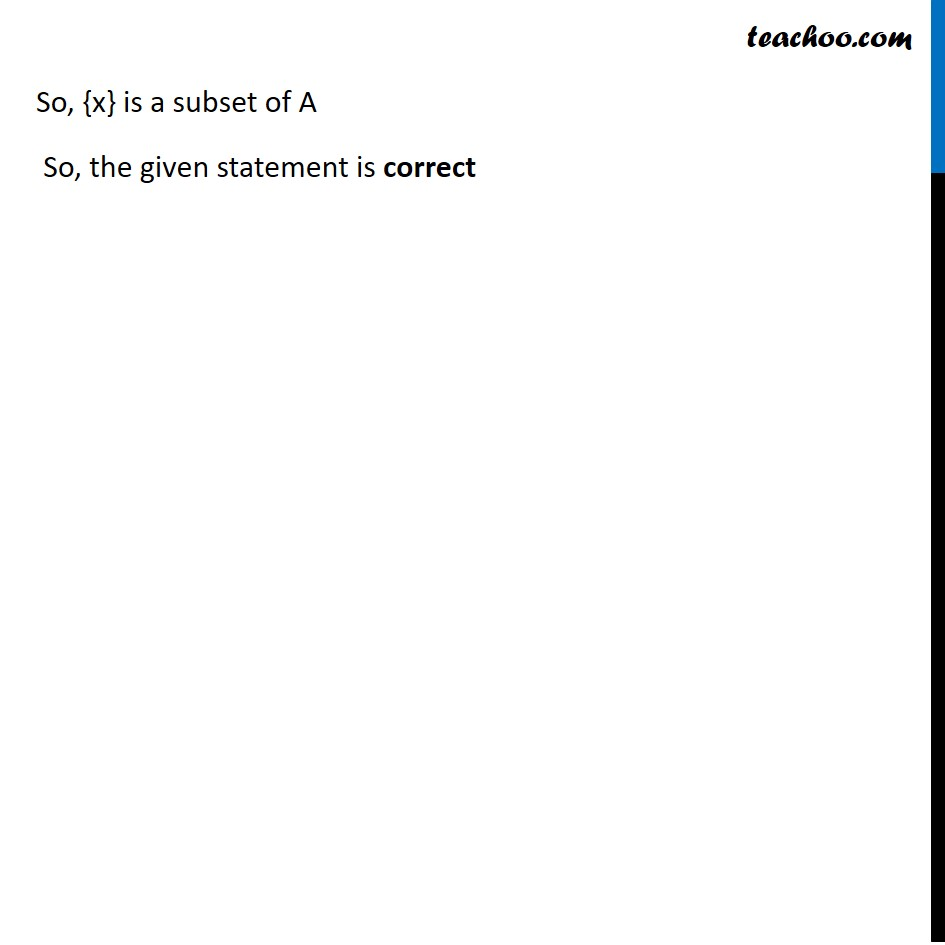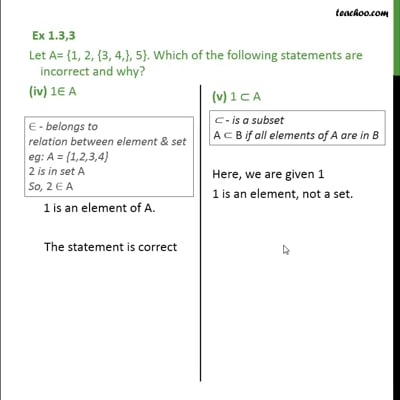This video is only available for Teachoo black users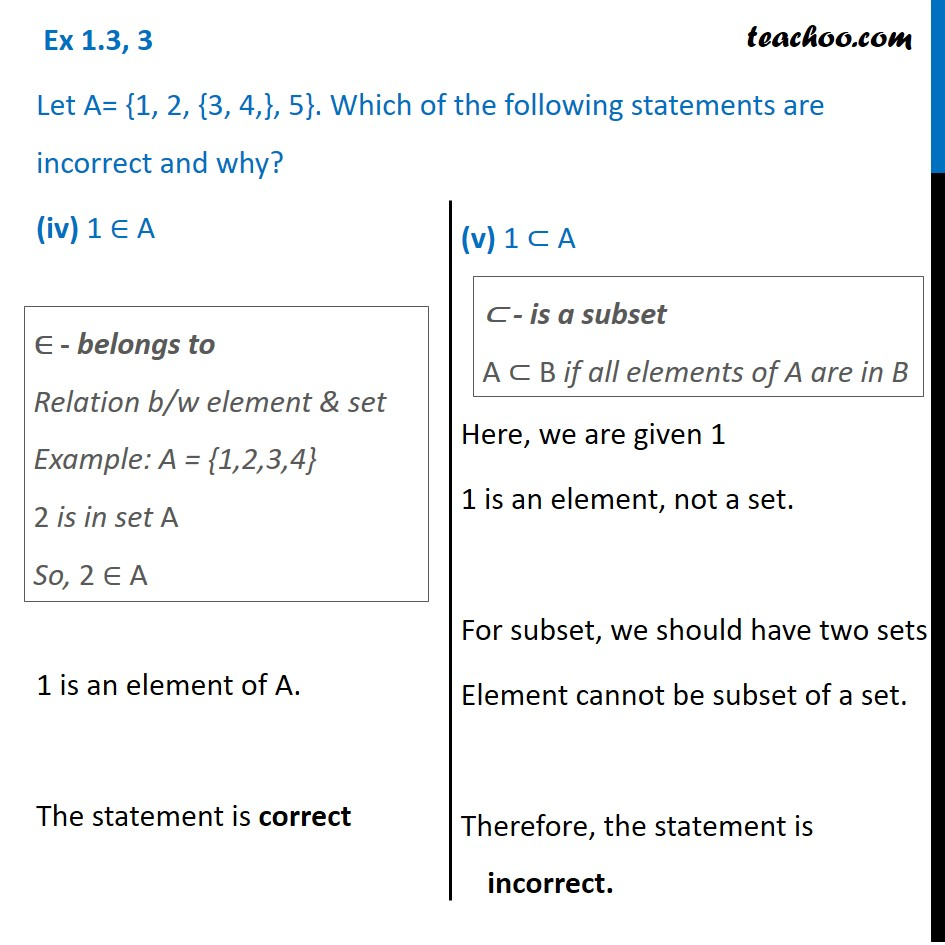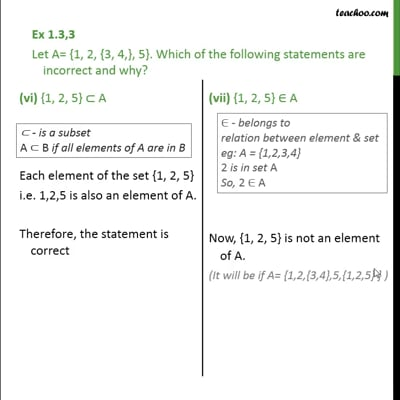This video is only available for Teachoo black users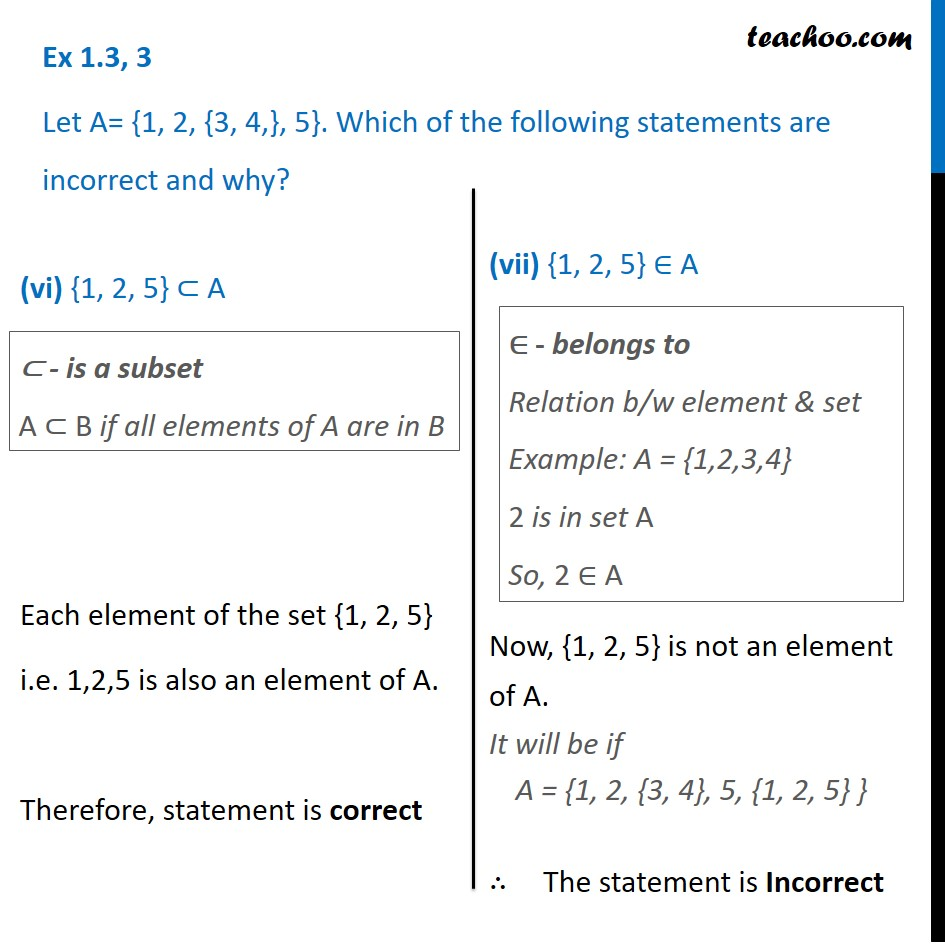This video is only available for Teachoo black users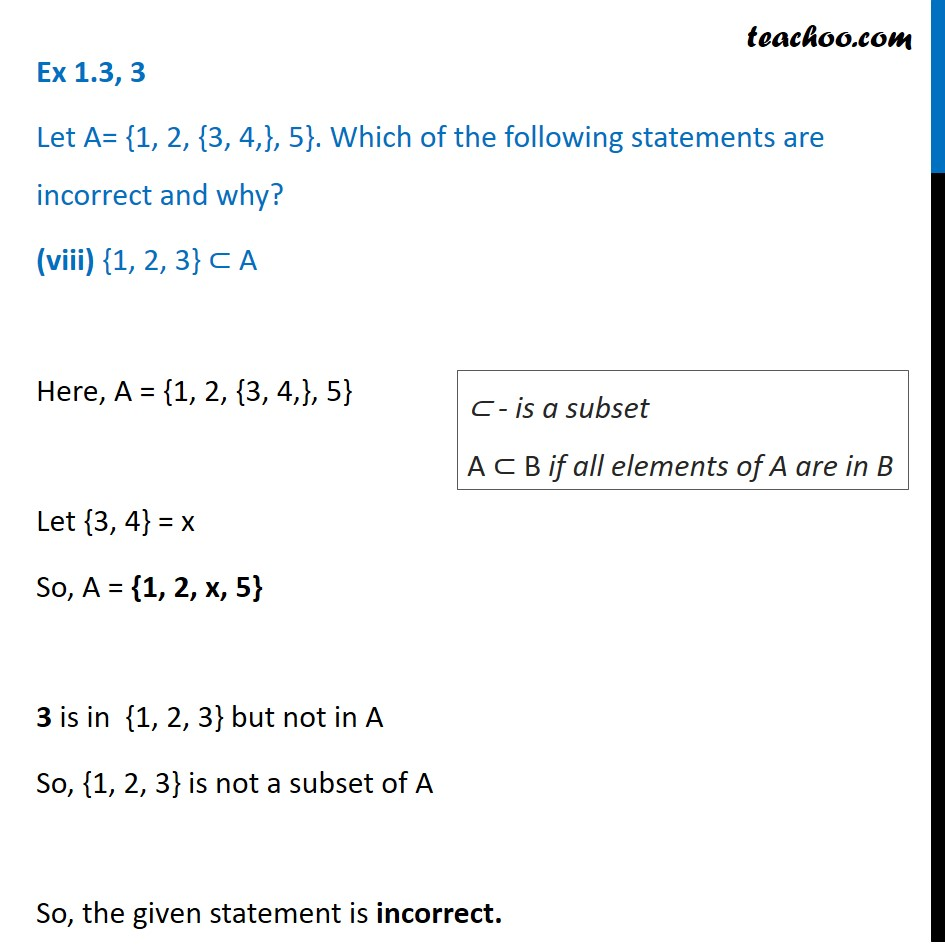This video is only available for Teachoo black users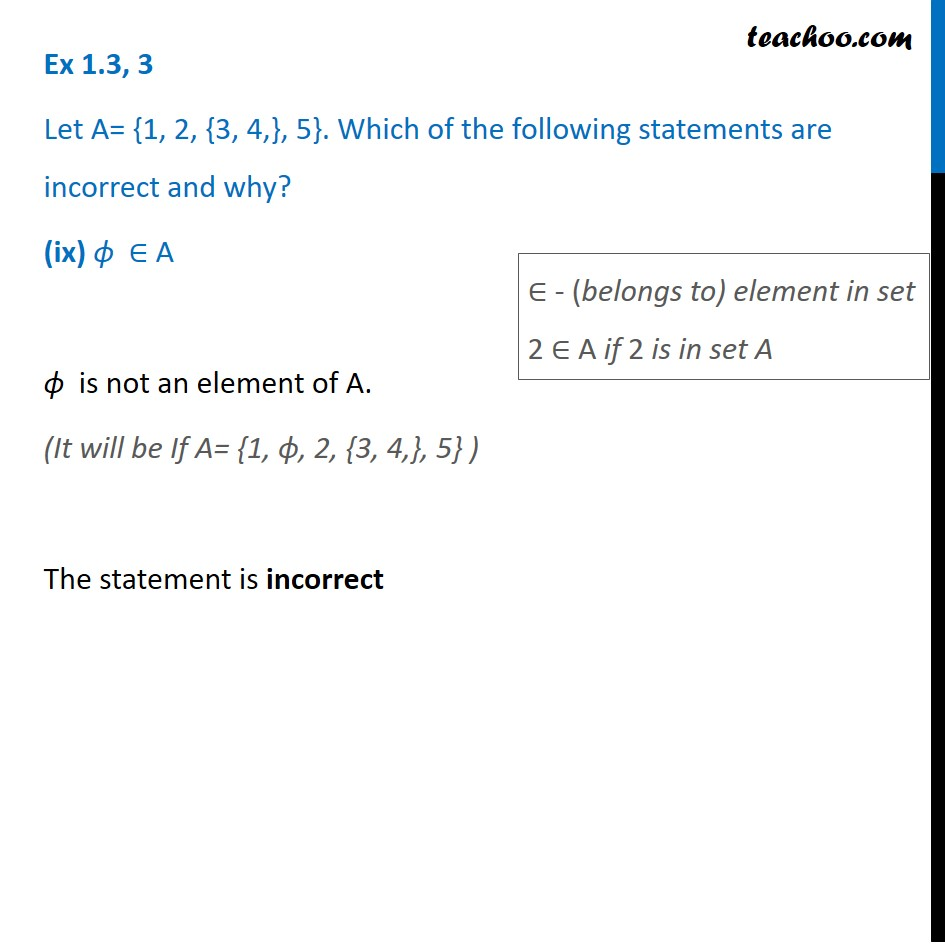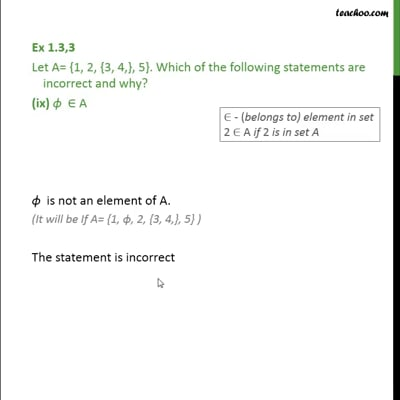This video is only available for Teachoo black users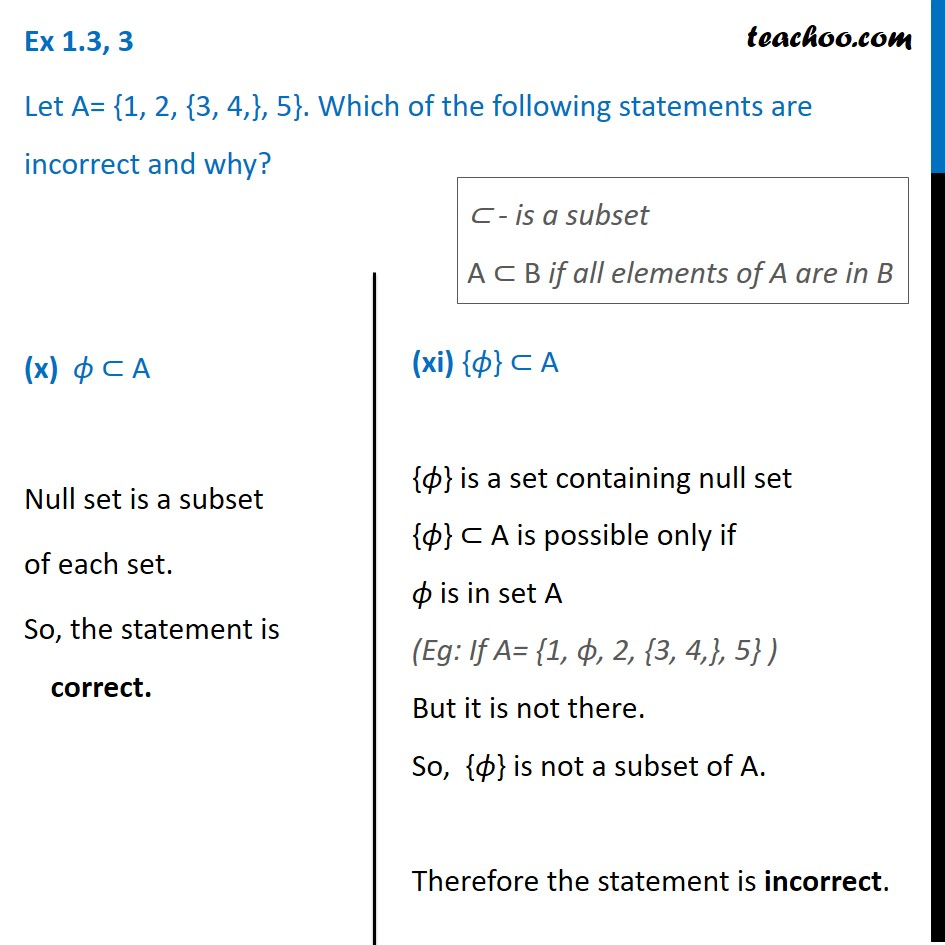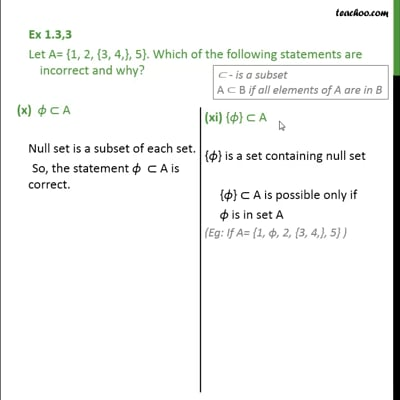This video is only available for Teachoo black users

### Transcript

Ex 1.3, 3 Let A = {1, 2, {3, 4}, 5}. Which of the following statements are incorrect and why? (i) {3, 4} ⊂ A Here, A = {1, 2, {3, 4,} 5} Let {3, 4} = x So, A = {1, 2, x, 5} 3, 4 is in {3, 4} but not in A So, {3, 4} is not a subset of A So, the given statement is incorrect. Ex 1.3, 3 Let A = {1, 2, {3, 4}, 5}. Which of the following statements are incorrect and why? (i) {3, 4} ⊂ A Here, A = {1, 2, {3, 4,} 5} Let {3, 4} = x So, A = {1, 2, x, 5} 3, 4 is in {3, 4} but not in A So, {3, 4} is not a subset of A So, the given statement is incorrect. ⊂ - is a subset A ⊂ B if all elements of A are in B Ex 1.3, 3 Let A= {1, 2, {3, 4,}, 5}. Which of the following statements are incorrect and why? (ii) {3, 4} ∈ A Let {3, 4} = x A = {1, 2, {3, 4,}, 5} So, A = {1, 2, x, 5} Now, x is in set A So, x ∈ A ∴ The statement is correct ∈ - belongs to relation between element & set eg: A = {1, 2, 3, 4} 2 is in set A So, 2 ∈ A Ex 1.3, 3 Let A= {1, 2, {3, 4}, 5}. Which of the following statements are incorrect and why? (iii) {{3, 4}}⊂ A {{3, 4}} is a set containing {3, 4} as an element Let {3, 4} = x So, {{3, 4}} = {x} Also, A = {1, 2, {3, 4}, 5} So, A = {1, 2, x, 5} So, x is in {x} and also in A ⊂ - is a subset A ⊂ B if all elements of A are in B So, {x} is a subset of A So, the given statement is correct Ex 1.3, 3 Let A= {1, 2, {3, 4,}, 5}. Which of the following statements are incorrect and why? (iv) 1 ∈ A 1 is an element of A. The statement is correct ∈ - belongs to Relation b/w element & set Example: A = {1,2,3,4} 2 is in set A So, 2 ∈ A ⊂ - is a subset A ⊂ B if all elements of A are in B (v) 1 ⊂ A Here, we are given 1 1 is an element, not a set. For subset, we should have two sets Element cannot be subset of a set. Therefore, the statement is incorrect. Ex 1.3, 3 Let A= {1, 2, {3, 4,}, 5}. Which of the following statements are incorrect and why? (vi) {1, 2, 5} ⊂ A Each element of the set {1, 2, 5} i.e. 1,2,5 is also an element of A. Therefore, statement is correct ⊂ - is a subset A ⊂ B if all elements of A are in B ∈ - belongs to Relation b/w element & set Example: A = {1,2,3,4} 2 is in set A So, 2 ∈ A (vii) {1, 2, 5} ∈ A Now, {1, 2, 5} is not an element of A. It will be if A = {1, 2, {3, 4}, 5, {1, 2, 5} } ∴ The statement is Incorrect Ex 1.3, 3 Let A= {1, 2, {3, 4,}, 5}. Which of the following statements are incorrect and why? (viii) {1, 2, 3} ⊂ A Here, A = {1, 2, {3, 4,}, 5} Let {3, 4} = x So, A = {1, 2, x, 5} 3 is in {1, 2, 3} but not in A So, {1, 2, 3} is not a subset of A So, the given statement is incorrect. ⊂ - is a subset A ⊂ B if all elements of A are in B Ex 1.3, 3 Let A= {1, 2, {3, 4,}, 5}. Which of the following statements are incorrect and why? (ix) ϕ ∈ A ϕ is not an element of A. (It will be If A= {1, ϕ, 2, {3, 4,}, 5} ) The statement is incorrect Ex 1.3, 3 Let A= {1, 2, {3, 4,}, 5}. Which of the following statements are incorrect and why? (ix) ϕ ∈ A ϕ is not an element of A. (It will be If A= {1, ϕ, 2, {3, 4,}, 5} ) The statement is incorrect ∈ - (belongs to) element in set 2 ∈ A if 2 is in set A Ex 1.3, 3 Let A= {1, 2, {3, 4,}, 5}. Which of the following statements are incorrect and why? (x) ϕ ⊂ A Null set is a subset of each set. So, the statement is correct. ⊂ - is a subset A ⊂ B if all elements of A are in B (xi) {ϕ} ⊂ A {ϕ} is a set containing null set {ϕ} ⊂ A is possible only if ϕ is in set A (Eg: If A= {1, ϕ, 2, {3, 4,}, 5} ) But it is not there. So, {ϕ} is not a subset of A. Therefore the statement is incorrect.